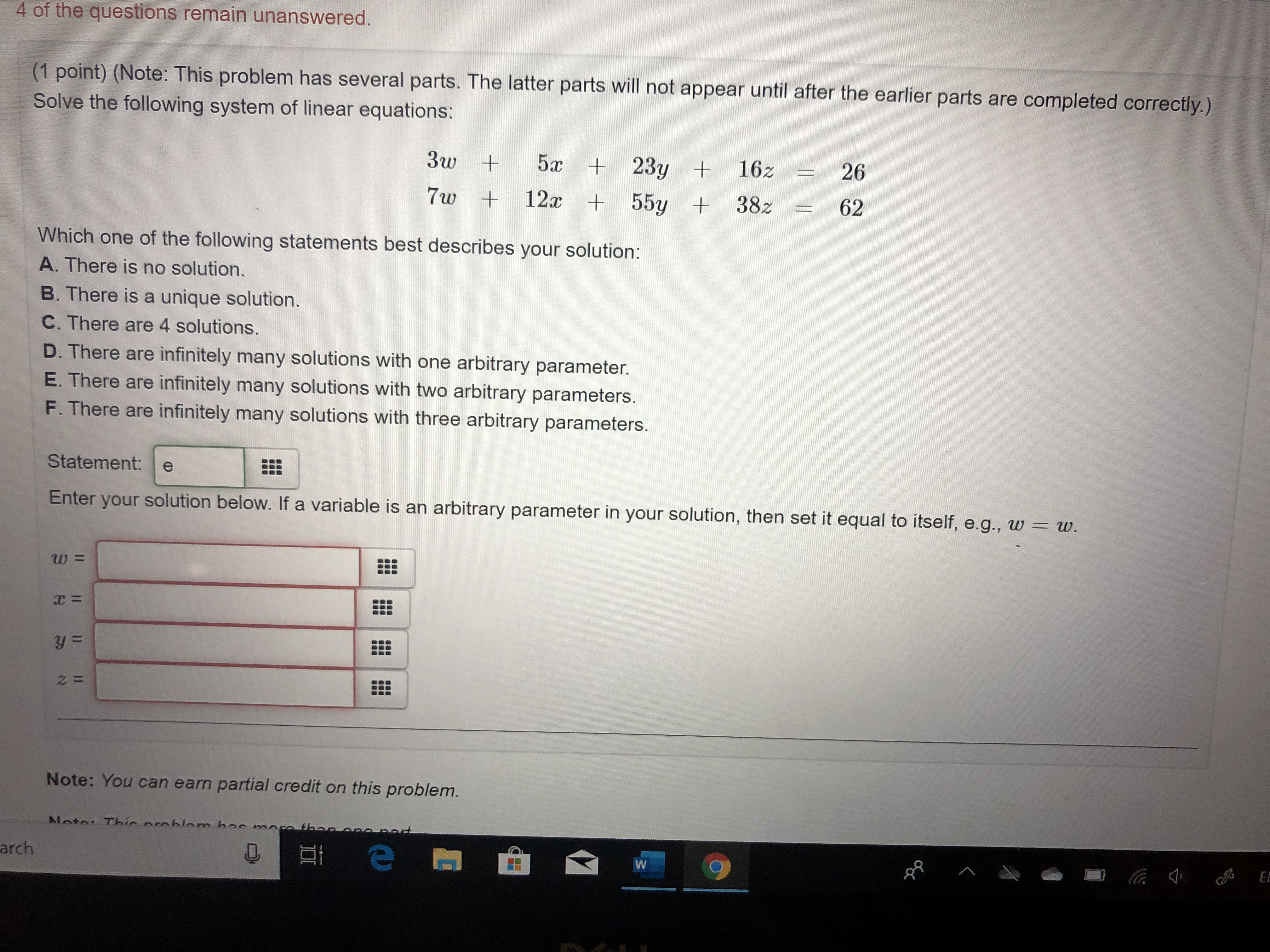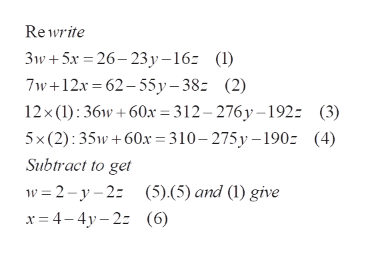# 4 of the questions remain unanswered.(1 point) (Note: This problem has several parts. The latter parts will not appear until after the earlier parts are completed correctly.)Solve the following system of linear equations:5x +23y +3w16226+12x +55y +38z627wWhich one of the following statements best describes your solution:A. There is no solution.B. There is a unique solution.C. There are 4 solutions.D. There are infinitely many solutions with one arbitrary parameter.E. There are infinitely many solutions with two arbitrary parameters.F. There are infinitely many solutions with three arbitrary parameters.Statement:esolution below. If a variable is an arbitrary parameter in your solution, then set it equal to itself, e.g., w =Enterw.yourC=y=z =Note: You can earn partial credit on this problem.Nata Thic nrahlom baro thanotElarchWaaaan

Question
43 views

If the variable is an arbitrary parameter in your solution, then set it equal to itself, e.g., w = whelp_outlineImage Transcriptionclose4 of the questions remain unanswered. (1 point) (Note: This problem has several parts. The latter parts will not appear until after the earlier parts are completed correctly.) Solve the following system of linear equations: 5x +23y + 3w 162 26 +12x +55y +38z 62 7w Which one of the following statements best describes your solution: A. There is no solution. B. There is a unique solution. C. There are 4 solutions. D. There are infinitely many solutions with one arbitrary parameter. E. There are infinitely many solutions with two arbitrary parameters. F. There are infinitely many solutions with three arbitrary parameters. Statement: e solution below. If a variable is an arbitrary parameter in your solution, then set it equal to itself, e.g., w = Enter w. your C= y= z = Note: You can earn partial credit on this problem. Nata Thic nrahlom ba ro thano t El arch W aa aan fullscreen
check_circle

Step 1

The problem concerns finding solutions (if any, and the number of such solutions) for 2 equations in 4 unknowns ,

Step 2

There are 2 equations , which are independent (one is not a multiple of the other). so , they are definitley consistent; To findi all the solutions, proceed as shown. Observe t...help_outlineImage TranscriptioncloseRe write 3w+5x 26-23y-16 () 7w+12x-62-55y-38z (2) 12x (1) 36w60x = 312-276y-192- (3) 5x (2): 35w+60x= 310-275y -190 (4) Subtract to get w 2-y2:(5).(5) and (1) give x 4-4 (6) fullscreen

### Want to see the full answer?

See Solution

#### Want to see this answer and more?

Solutions are written by subject experts who are available 24/7. Questions are typically answered within 1 hour.*

See Solution
*Response times may vary by subject and question.
Tagged in

### Math